﻿﻿ 波涛高级设计师季玲羽-上海波涛装饰集团海安公司
﻿•金石蓝郡

•天澜湾

•万达 海之心

•万达 海之心

•万达 海之心

•华新御园

•星湖湾

•苏中尚城

•金石蓝郡

•恒盛新座

•公园印象

•海州阳光城

•华新御园

•文顺花苑

•文顺花苑

•书香园

•文顺花苑

•华新一品

•黎明润景

•文顺花苑

•盛世名门

•金石蓝郡

•奥体尚府

•书香园

•书香园

•万豪

•书香园

•书香园

•书香园

•书香园

•书香园

•晨朗

•恒盛新座

•华新一品

•优景美地

•东港

•金水湾

•吉祥水岸

•盛世名门

•曙光澜桥

•书香园

•天澜湾

•界墩花苑

•曙光澜桥

•苏中尚城

•盛世名门

•金水湾

•书香园

•清水园

•黎明润景

•金石翡丽郡

•华新御园

•吉祥水岸

•万豪国际

•海洲新城

•天澜湾

•奥体尚府

•万豪

•富御广场

•优景美地

•华新一品

•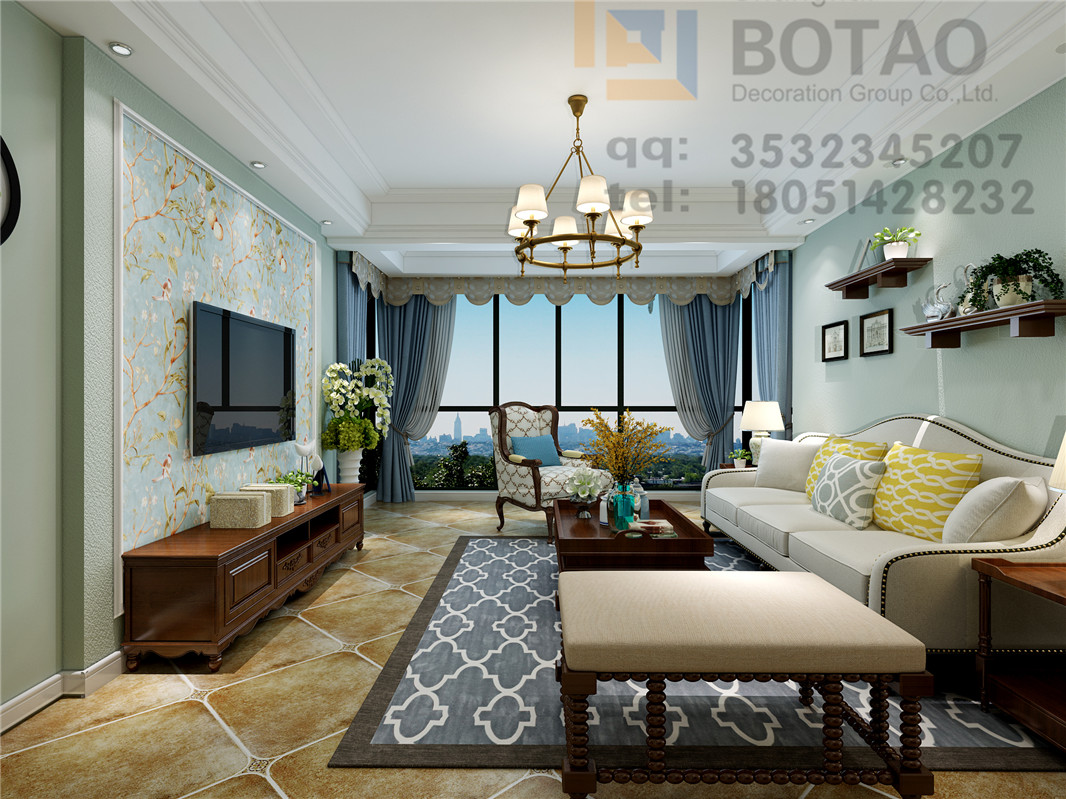天澜湾

•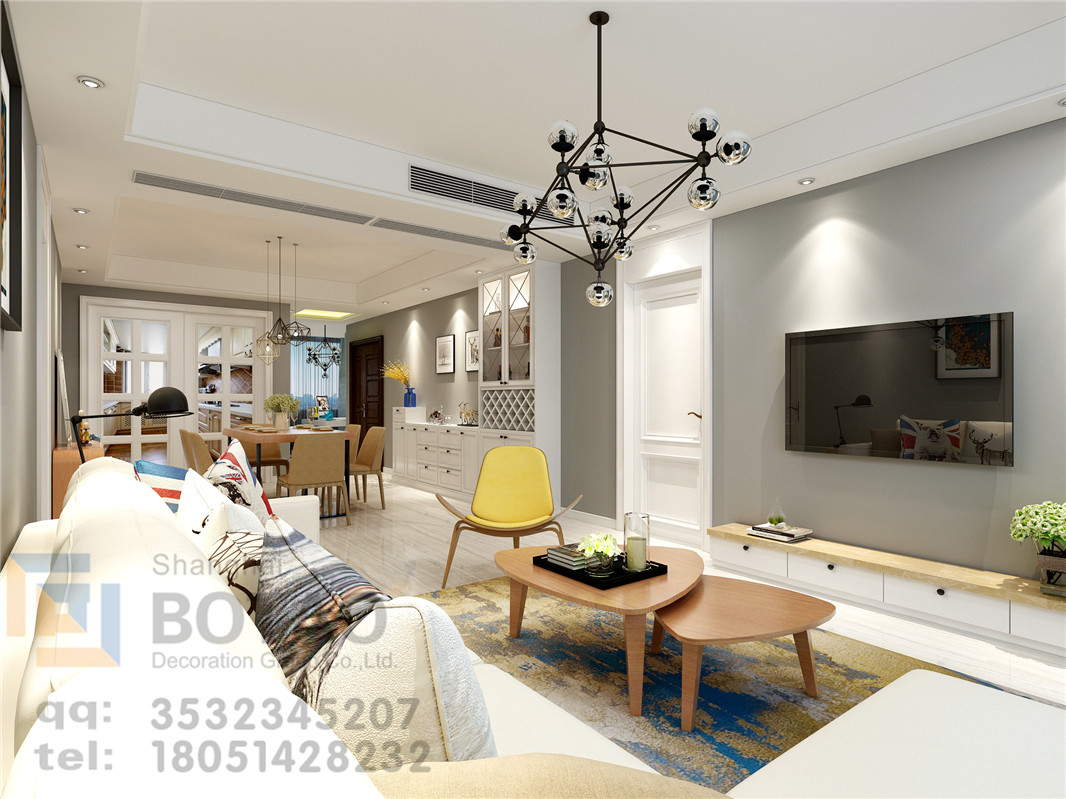东港国际

•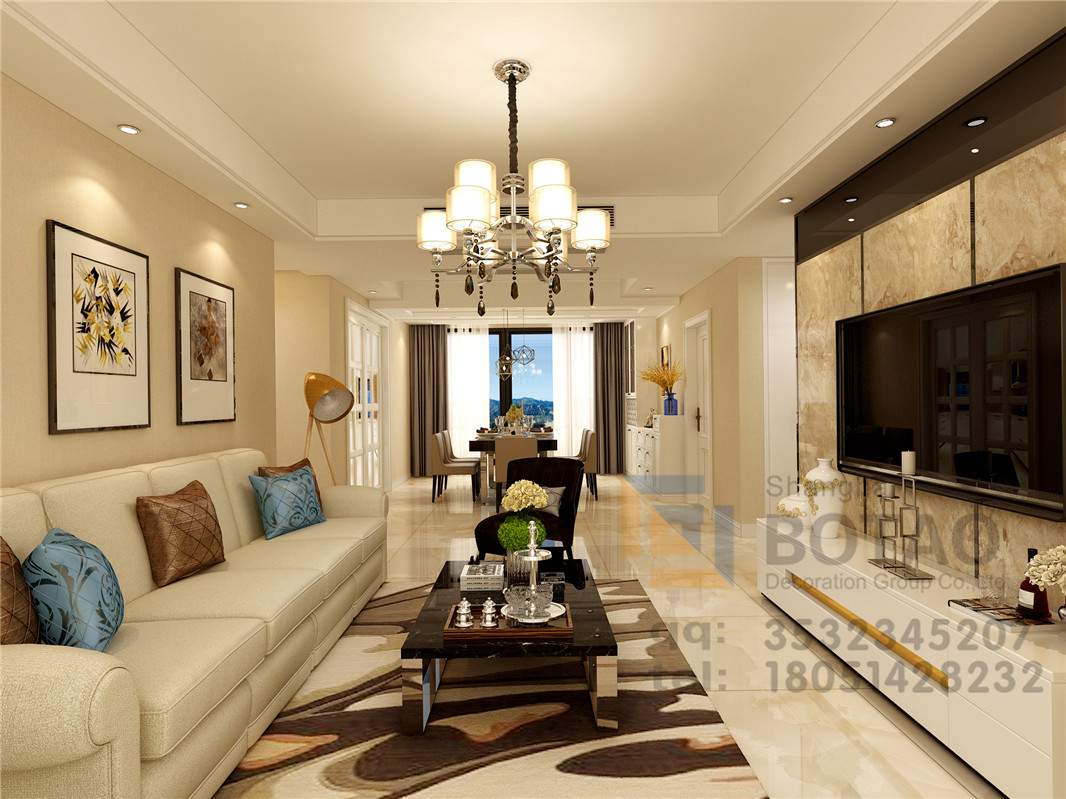香溢花城

•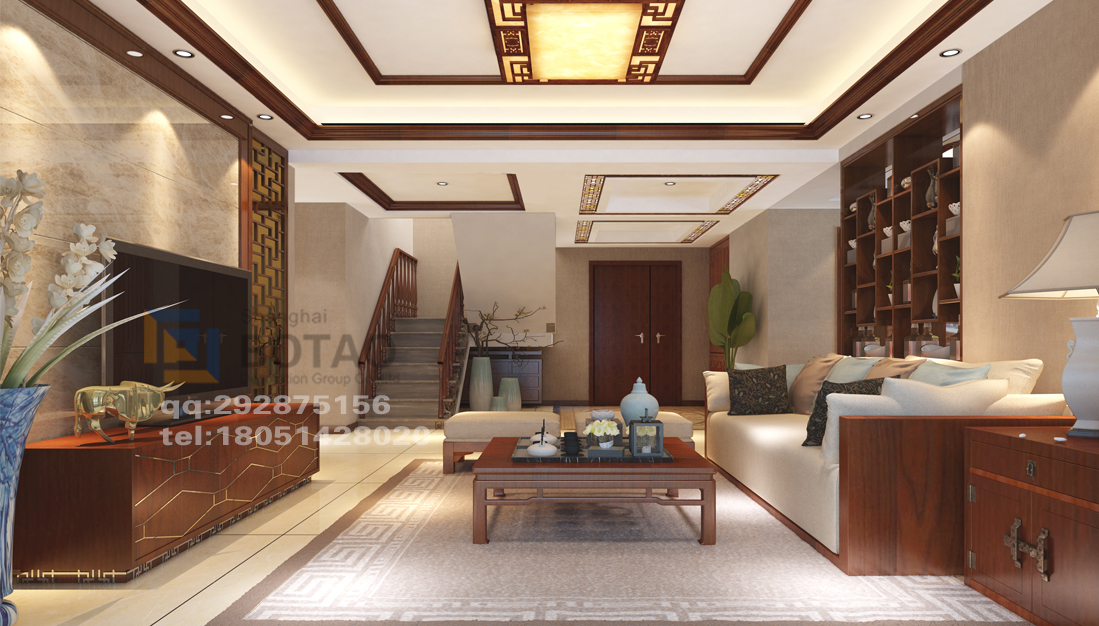苏中尚城

•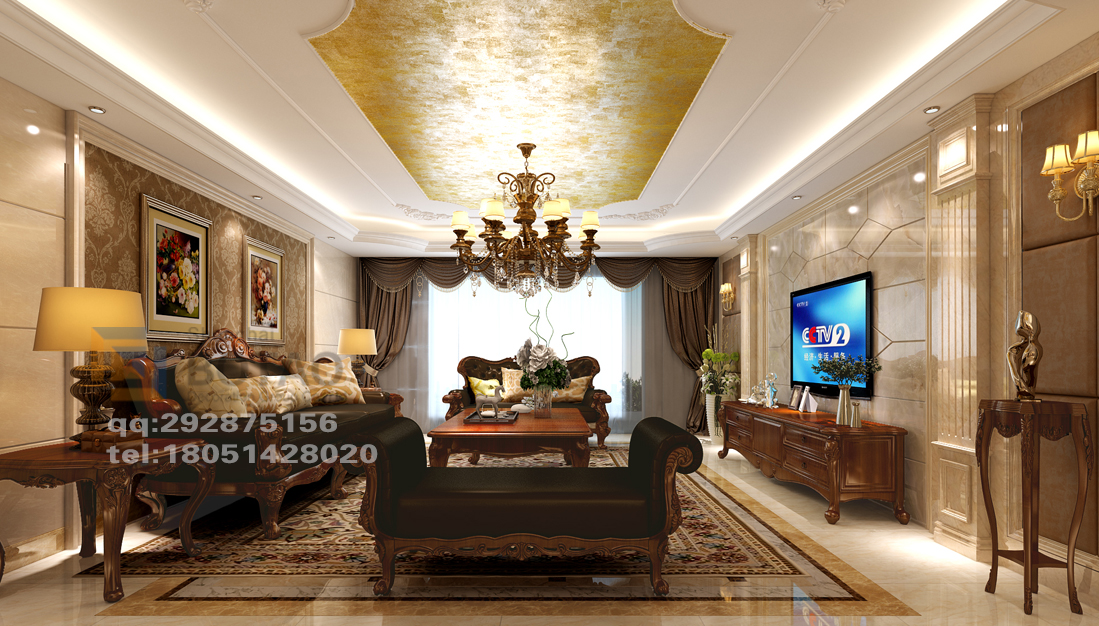天澜湾

•凯顺瑞城

•苏中尚城

•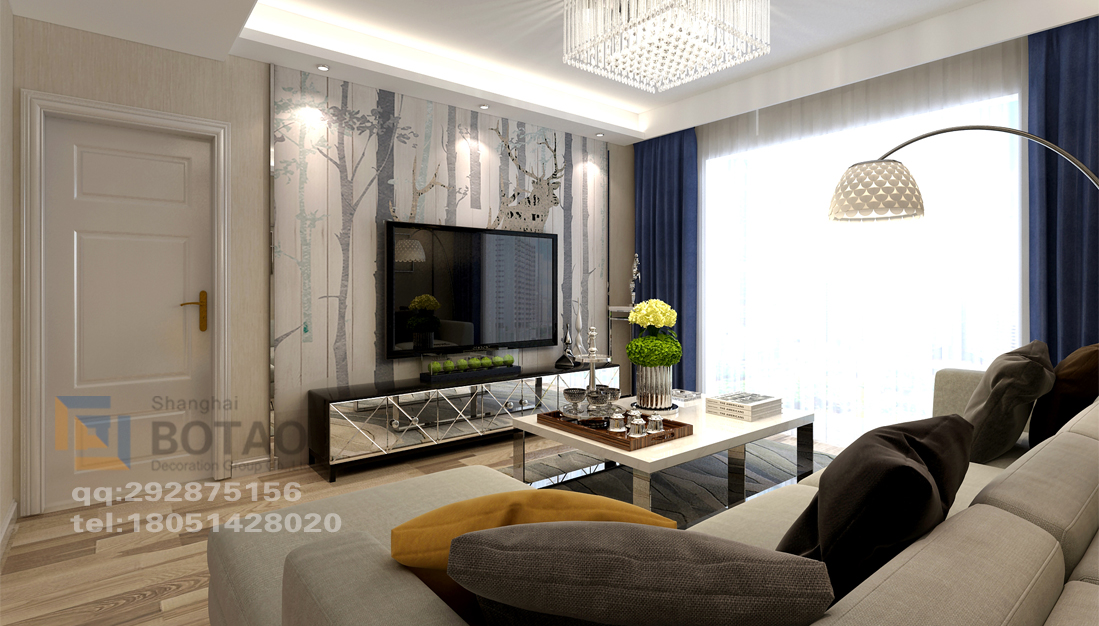华新一品

•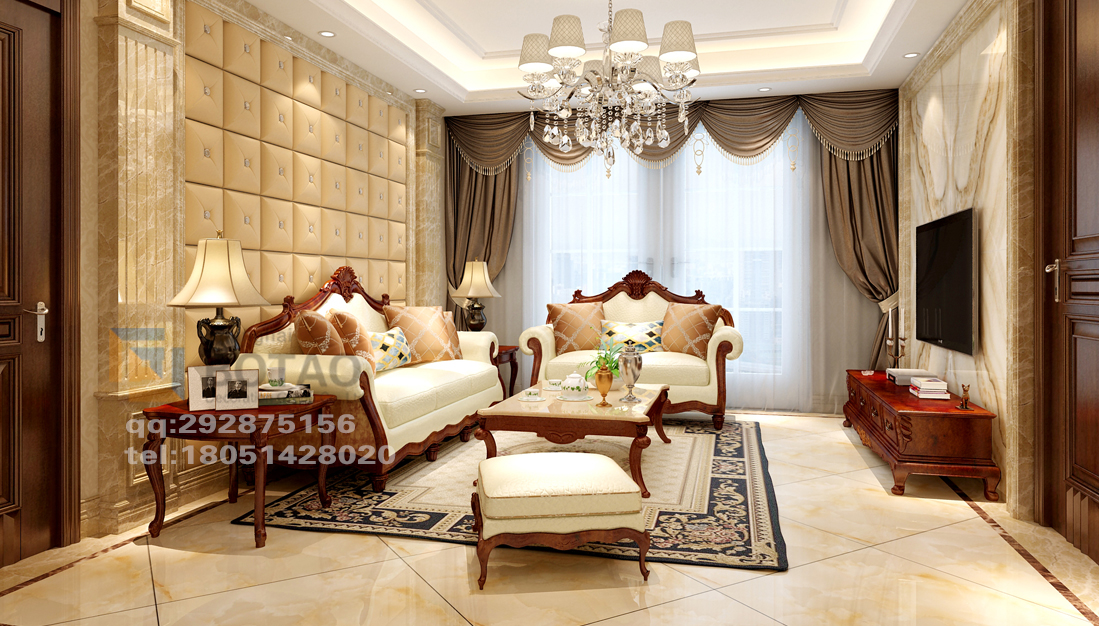东港国际

•人才公寓

•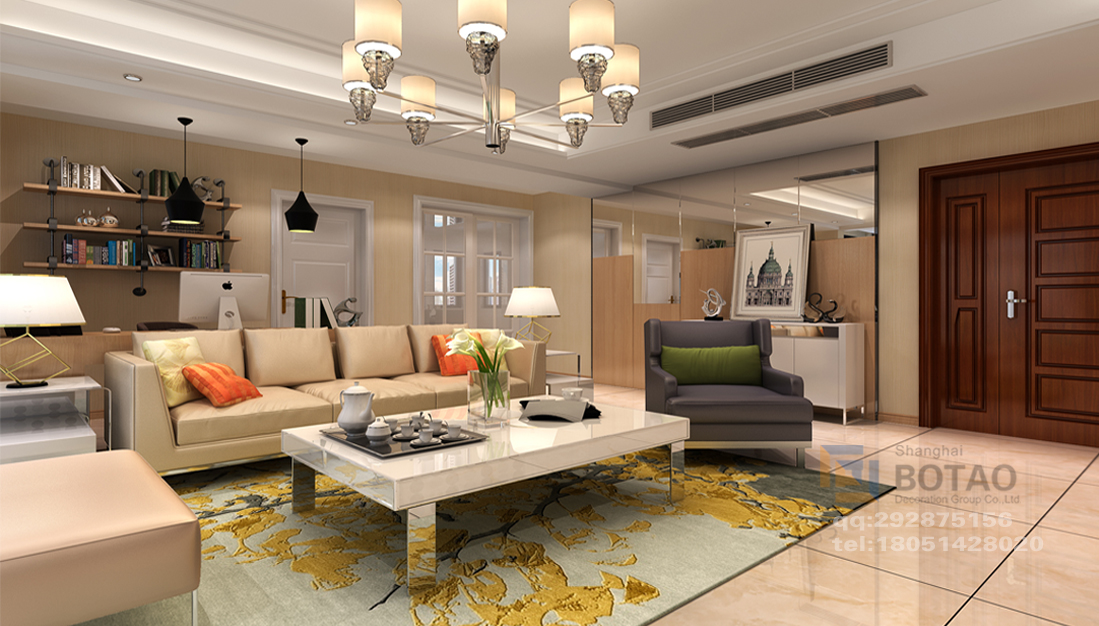华新一品·御园

•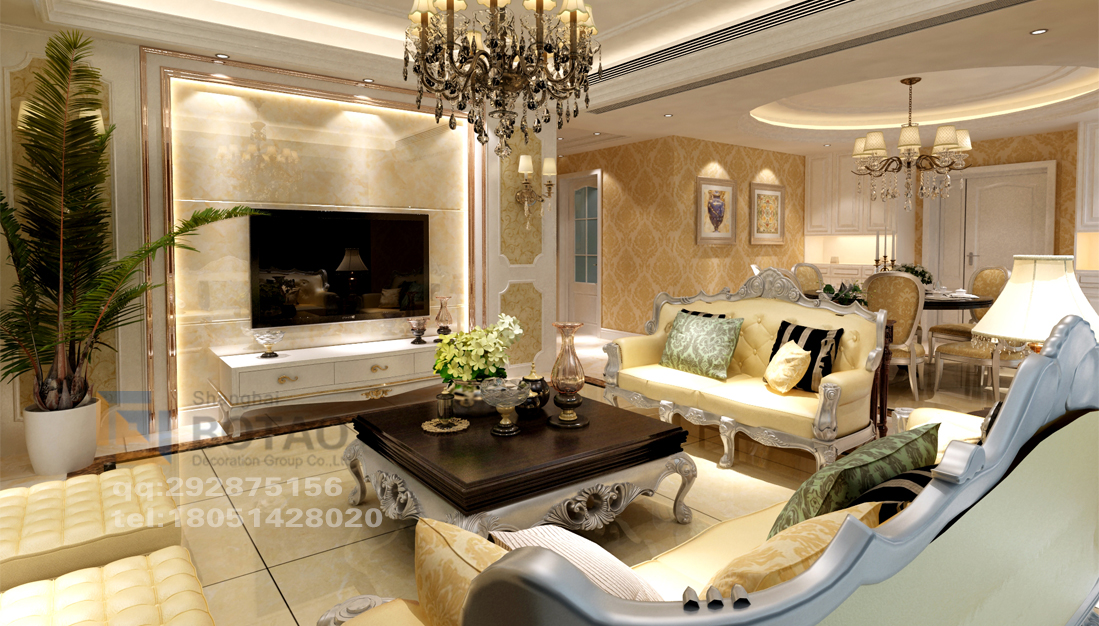苏中天澜湾

•苏中尚城

•华新一品·御元

•苏中尚城

•苏中天澜湾

•华新一品·御元

•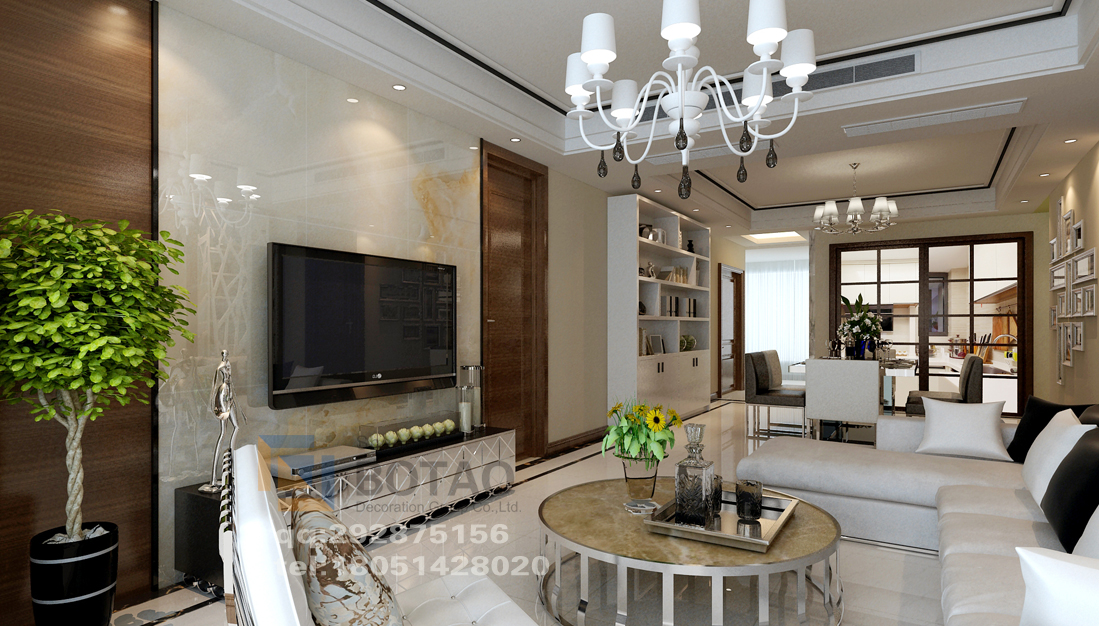东港国际

•万豪国际

本方案是围绕现代简约为主题，适合于30岁左右的三口之家居住。以简洁明快的设计风格为主调，简洁和实用是现代简约风格的基本。全面考虑，在总体布局上方面尽量满足业主生活的需求，主要装修材料以壁纸、硬包为主，以硬包、壁纸的朴素大方来装饰墙面的景点。更体现现代简约的之感。创造一个温馨，健康的家庭。
•华府天地

•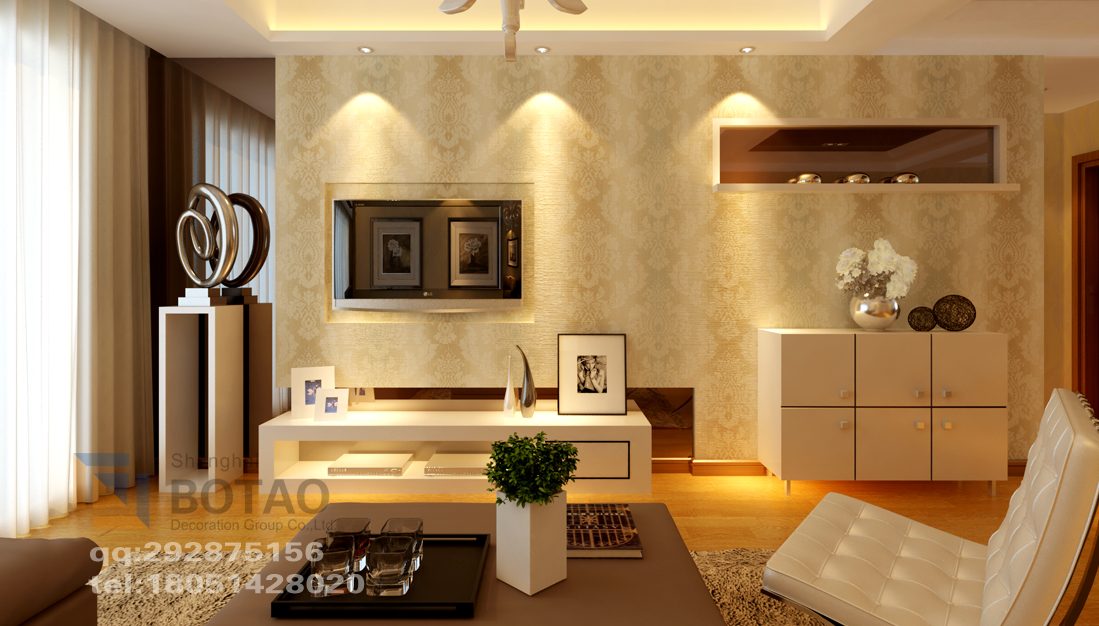华府天地

•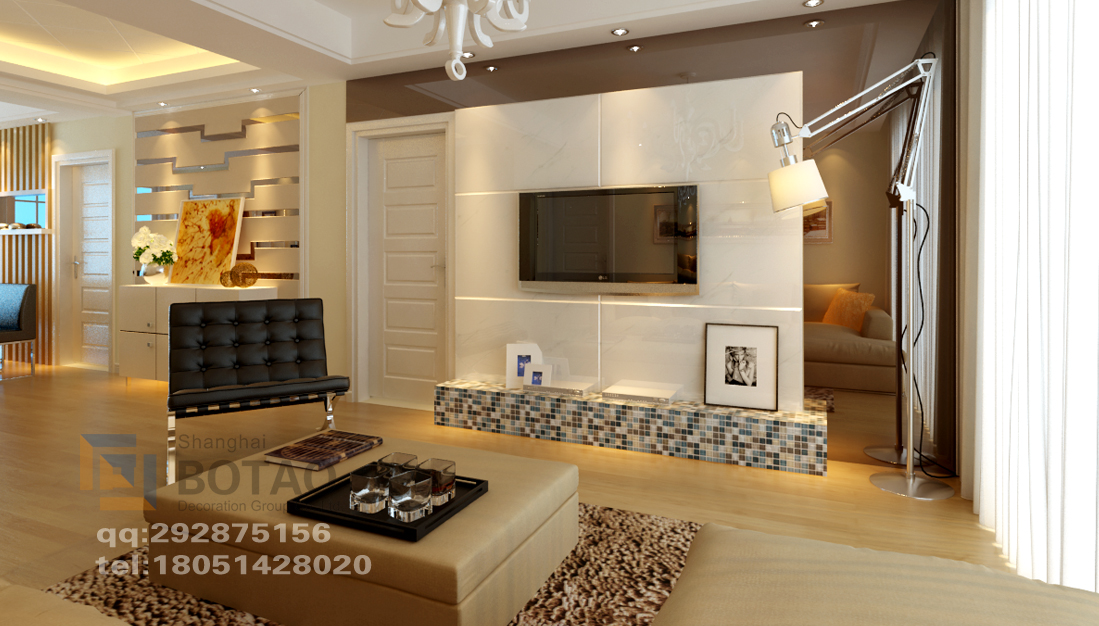华府天地

•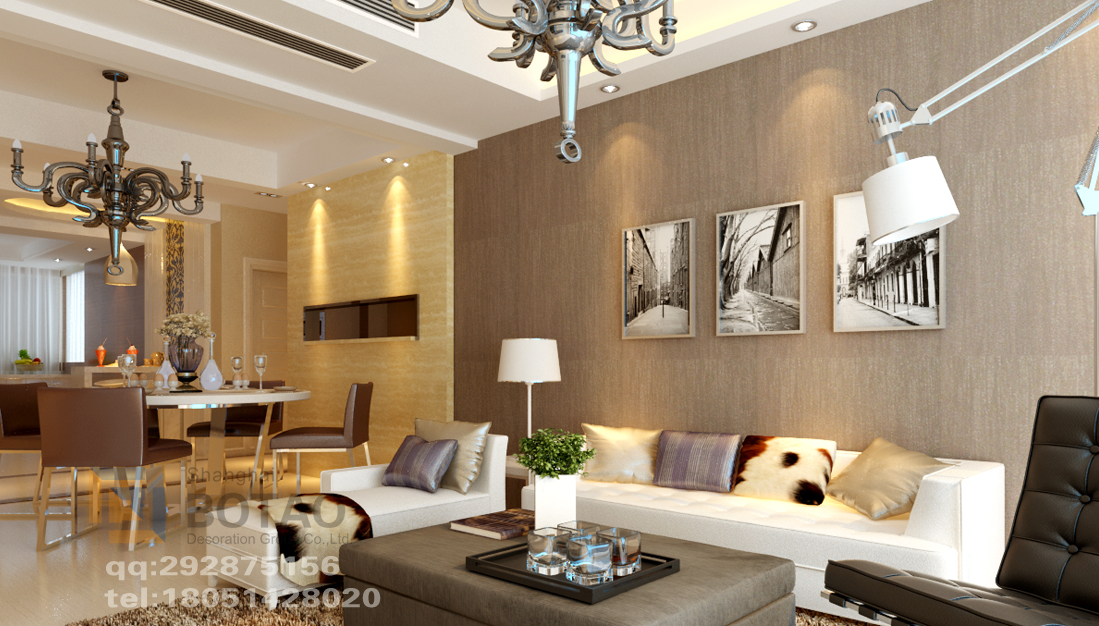华府天地

•华府天地

•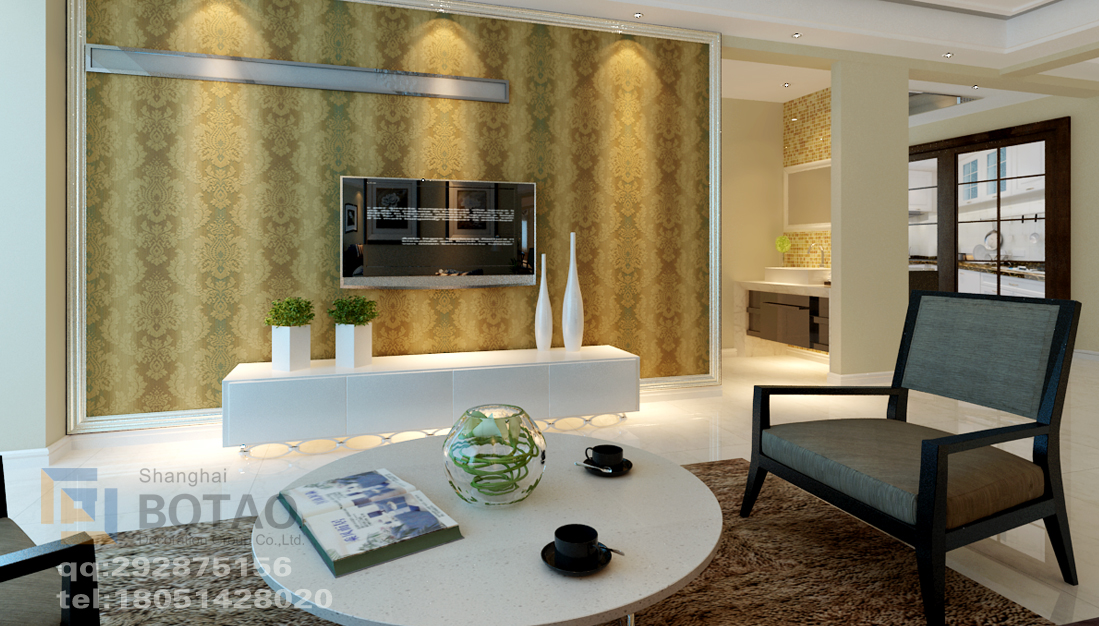富安 富御广场

•富安 富御广场

•奥体尚府

•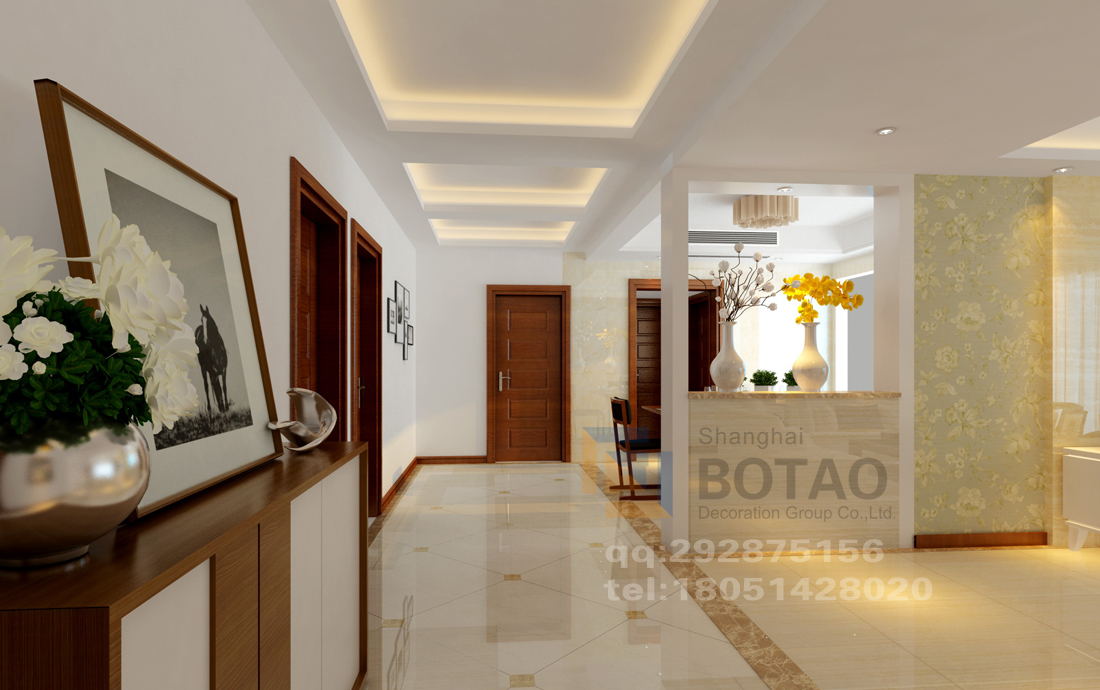城南花苑

•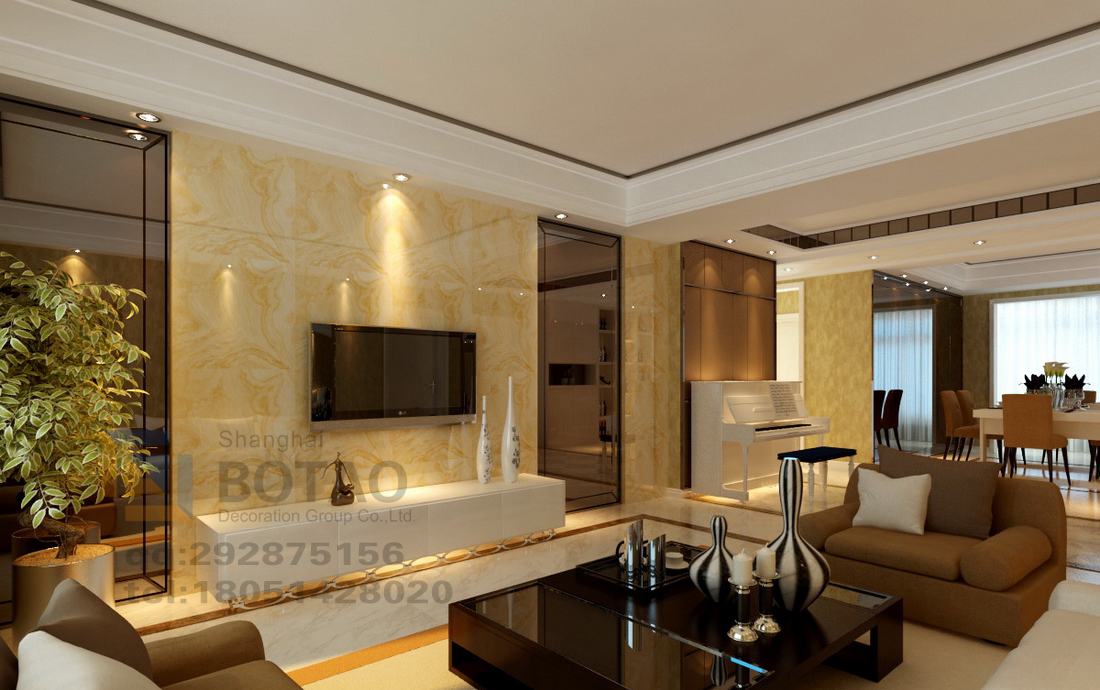万豪国际

﻿